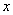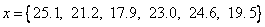| Next >

## Beginning Data Science - Learning From Data

In this tutorial, you will learn simple statistical concepts about central tendency (Mean, Median, and Mode) and variation of data (Range, Variance and Standard Deviation).

Suppose you run for 100-meter sprint for 6 times and you measure your running time by a stopwatch (in second, up to 1 digit significant). The running time is a set of data that can be put into one variable name= running time in seconds. For example, your data is like thisWhat you can learn from this data? Here is a simple statistics of your running time:

• The average (or arithmetic mean) of your running time is 21.9 seconds
• The mid value or median of your running time is 22.1 seconds
• The maximum running time is 25.1 seconds and the minimum running time is 17.9 seconds. Now you can compare your record with one of the fastest world record in 2002 of 9.78 seconds. Well, you can say that you just run because you like the sport and hobby, not as a professional athlete.
• The variance of your running time is 8.2 square-seconds and the standard deviation is 2.9 seconds.

Click the following topics for more detail information regarding this simple statistics

Central Tendency - learn about mean, median and mode
Variation of Data - learn about Range, Interquartile range and Variance, and standard deviation
Excel function reference related to this tutorial are listed here.